Download Presentation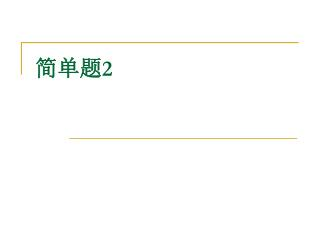# 简单题 2 - PowerPoint PPT Presentation

%ld",sum); if(j<=2) s*=7; else s*=8; sum+=s;} printf("
sum=%ld",sum); }. 四位数. 求具有 abcd=(ab+cd)*(ab+cd) 性质的四位数I am the owner, or an agent authorized to act on behalf of the owner, of the copyrighted work described.
Download Presentation## 简单题 2

An Image/Link below is provided (as is) to download presentation

Download Policy: Content on the Website is provided to you AS IS for your information and personal use and may not be sold / licensed / shared on other websites without getting consent from its author.While downloading, if for some reason you are not able to download a presentation, the publisher may have deleted the file from their server.

- - - - - - - - - - - - - - - - - - - - - - - - - - E N D - - - - - - - - - - - - - - - - - - - - - - - - - -
Presentation Transcript### 简单题2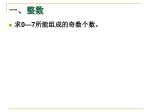• 求0—7所能组成的奇数个数。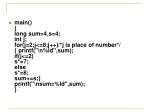main(){long sum=4,s=4;int j;for(j=2;j<=8;j++)/*j is place of number*/{ printf("\n%ld",sum);if(j<=2)s*=7;elses*=8;sum+=s;}printf("\nsum=%ld",sum);}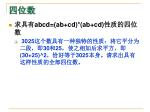• 求具有abcd=(ab+cd)*(ab+cd)性质的四位数
• 3025这个数具有一种独特的性质：将它平分为二段，即30和25，使之相加后求平方，即(30+25)^2，恰好等于3025本身。请求出具有这样性质的全部四位数。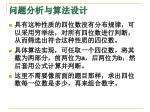• 具有这种性质的四位数没有分布规律，可以采用穷举法，对所有四位数进行判断，从而筛选出符合这种性质的四位数。
• 具体算法实现，可任取一个四位数，将其截为两部分，前两位为a，后两位为b，然后套用公式计算并判断。
• 这里不需要像前面的题目那样，求出四位数每一位数是多少，再来组合数字。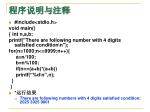• #include<stdio.h>

void main()

{ int n,a,b;

printf("There are following number with 4 digits satisfied condition\n");

for(n=1000;n<=9999;n++){

a=n/100;

b=n%100;

if(n==(a+b)*(a+b))

printf(“%d\n”,n);

}

}

• *运行结果
• There are following numbers with 4 digits satisfied condition: 2025 3025 9801• 编程验证“大于1000的奇数其平方与1的差是8的倍数”。
• *问题分析与算法设计
• 数学证明：
• 设这个奇数为999+2a，a为大于或等于1的整数。则
• (999+2a)^2-1=4a^2+3996a+998000=4(a^2+999a)+998000
• 后半部分是8的倍数，而前半部分，不管a是奇数还是偶数，a^2+999a都是是一个偶数，那么再在前面乘以4的话，其结果一定是8的倍数。
• 我们可以编写程序验证它。
• 题目中给出的处理过程很清楚，算法不需要特殊设计。可以按照题目的叙述直接进行验证(程序中仅验证到3000)。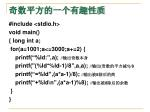#include <stdio.h>

void main()

{ long int a;

for(a=1001;a<=3000;a+=2) {

printf(“%ld:”,a); //输出奇数本身

printf("(%ld*%ld-1)/8",a,a); //输出(奇数的平方减1)/8

printf(“=%ld”,(a*a-1)/8); //输出被8除后的商

printf(“+%ld\n”,(a*a-1)%8); //输出被8除后的余数

}

}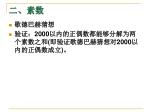• 歌德巴赫猜想
• 验证：2000以内的正偶数都能够分解为两个素数之和(即验证歌德巴赫猜想对2000以内的正偶数成立)。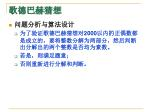• 问题分析与算法设计
• 为了验证歌德巴赫猜想对2000以内的正偶数都是成立的，要将整数分解为两部分，然后判断出分解出的两个整数是否均为素数。
• 若是，则满足题意；
• 否则重新进行分解和判断。#include <stdio.h>

#include <math.h>

void main()

{ bool isprime(int n);

int i,n;

for(i=4;i<=2000;i+=2) {

for(n=2;n<i;n++) // {

if(isprime(n)&&isprime(i-n)) {

printf("%d=%d+%d\n",i,n,i-n);

break;

}

}

} }• “1898–要发就发”。
• 请将不超过1993的所有素数从小到大排成第一行，第二行上的每个素数都等于它右肩上的素数之差。
• 编程求出：第二行数中是否存在这样的若干个连续的整数，它们的和恰好是1898？假好存在的话，又有几种这样的情况？
• 第一行：2 3 5 7 11 13 17……1979 1987 1993
• 第二行：1 2 2 4 2 4…… 8 6• 问题分析与算法设计
• 首先从数学上分析该问题： 假设第一行中的素数为n、n、n….n[i]、…第二行中的差值为m、m、m…m[j]…。其中m[j]为： m[j]=n[j+1]-n[j]。 则第二行连续N个数的和为： SUM=m+m+m+…+m[j] =(n-n)+(n-n)+(n-n)+…+(n[j+1]-n[j]) =n[j+1]-n
• 由此题目就变成了：在不超过1993的所有素数中是否存在这样两个素数，它们的差恰好是1898。若存在，则第二行中必有所需整数序列，其和恰为1898。 对等价问题的求解是比较简单的。 由分析可知，在素数序列中不必包含2，因为任意素数与2的差一定为奇数，所以不必考虑。#include <stdio.h>

#include <math.h>

#define NUM 320

bool isprime(int i);

void main()

{ int number[NUM];

int i,j,count=0;

printf("There are follwing primes seq in first row:\n");

for(j=0,i=3;i<=1993;i+=2)

if(isprime(i)) number[j++]=i;

for(j--;number[j]>1898;j--) {

for(i=0;number[j]-number[i]>1898;i++); {

if(number[j]-number[i]==1898)

printf("(%d)%3d,..,%d\n",++count,number[i],number[j]);

}

}

}• 百钱百鸡问题
• 爱因斯坦的数学题
• 三色球问题
• 若一个口袋中放有12个球，其中有3个红的，3个白的和6个黒的，问从中任取8个共有多少种不同的颜色搭配？• 问题分析与算法设计
• 设任取的红球个数为i，白球个数为j，则黒球个数为8-i-j，根据题意，红球和白球个数的取值范围都是0-3，在红球和白球个数确定的条件下，黒球个数取值应为8-i-j<=6。#include <stdio.h>

void main()

{ int i,j,count=0;

printf(" RED WHITE BLACK\n");

printf("…………………………………\n");

for(i=0;i<=3;i++) {

for(j=0;j<=3;j++) {

if((8-i-j)<=6)

printf("%2d: %4d %5d %6d\n",++count,i,j,8-i-j);

}

}

}• 马克思手稿中有一道趣味数学问题：
• 有30个人，其中有男人、女人和小孩，在一家饭馆吃饭花了50先令；每个男人花3先令，每个女人花2先令，每个小孩花1先令；
• 问男人、女人和小孩各有几人？• 问题分析与算法设计
• 设x,y,z分别代表男人、女人和小孩。
• 按题目的要求，可得到下面的方程： x+y+z=30 (1) 3x+2y+z=50 (2)
• 用方程程序求此不定方程的非负整数解，可先通过(2)-(1)式得： 2x+y=20 (3)
• 由(3)式可知，x变化范围是0-10。#include <stdio.h>

void main()

{ int x,y,z,count=0;

printf(" Men Women Children\n");

printf("…………………………………\n");

for(x=0;x<=10;x++) {

y=20-2*x;

z=30-x-y;

if(3*x+2*y+z==50)

printf("%2d: %2d %6d %7d\n",++count,x,y,z);

}

}• 某人有四张3分的邮票和三张5分的邮票，用这些邮票中的一张或若干张可以得到多少种不同的邮资？
• *问题分析与算法设计
• 将问题进行数学分析，不同张数和面值的邮票组成的邮资可用下列公式计算： S=3*i+5*j 其中i为3分邮票的张数，j为5分邮票的张数
• 按题目的要求，3分的邮票可以取0、1、2、3、4张，5分的邮票可以取0、1、2、3张。
• 采用穷举方法进行组合，可以求出这些不同面值不同张数的邮标组合后的邮资。#include<stdio.h>

int a;

void main()

{ int i,j,k,s,n=0;

for(i=0;i<=4;i++) {

for(j=0;j<=3;j++) {

s=i*3+j*5;

for(k=0;a[k];k++) {

if(s==a[k]) break;

}

if(!a[k]&&s) a[k]=s; n++;

}

}

printf("%d kinds:",n);

for(k=0;a[k];k++) printf("%d ",a[k]);

printf("\n");

}

• *运行结果

19 kinds: 5 10 15 3 8 13 18 6 11 16 21 9 14 19 24 12 17 22 27• 计算字符串中子串出现的次数#include "string.h"#include "stdio.h"main(){ char str1,str2,*p1,*p2;int sum=0;printf("please input two strings\n");scanf("%s%s",str1,str2);p1=str1;p2=str2;while(*p1!='\0'){ if(*p1==*p2) {while(*p1==*p2&&*p2!='\0') {p1++; p2++;} } else p1++; if(*p2=='\0') sum++; p2=str2;}printf("%d",sum);}• 将阿拉伯数字转换为罗马数字
• 将大于0小于1000的阿拉伯数字转换为罗马数字。
• 阿拉伯数字与罗马数字的对应关系如下：
• 1 2 3 4 5 6 7 8 9
• I II III IV V VI VII VIII IX
• 10 20 30 40 50 60 70 80 90
• X XX XXX XL L LX LXX LXXX XCC
• 100 200 300 400 500 600 700 800 900
• C CC CCC CD D DC DCC DCCC CM• 问题分析与算法设计
• 题目中给出了阿拉伯数字与罗马数字的对应关系，题中的数字转换实际上就是查表翻译。
• 即将整数的百、十、个位依次从整数中分解出来，查找表中相应的行后输出对应的字符。 *#include<stdio.h>

void main()

{ static char *a[]={“”,“I”,“II”,“III”,“IV”,“V”,“VI”,“VII”,“VIII”,“IX”, “”,“X”,“XX”,“XXX”,“XL”,“L”,“LX”,“LXX”,“LXXX”,“XCC”, “”,“C”,“CC”,“CCC”,“CD”,“D”,“DC”,“DCC”,“DCCC”,“CM” };

int n,t,i,m;

scanf(“%d”,&n); //输入整数

printf("%d=",n);

for(m=0,i=1000;m<3;m++,i/=10) {

t=(n%i)/(i/10); //从高位向低位依次取各位的数字

printf(“%s”,a[2-m][t]); //通过对照表翻译输出

}

printf("\n");

}

• *运行结果
• Please enter number:863 863=DCCCLXIII*思考题
• 输入正整数N，产生对应的英文数字符串并输出，例如：
• 1 ONE 2 TWO 3 THREE 10 TEN 11 ELEVEN 135 ONE HUNDRED THIRTY FIVE
• *问题分析与算法设计
• 注意英文表示数字的特点，调整二维数组的值。• 三天打鱼两天晒网
• 某人从1990年1月1日起开始“三天打鱼两天晒网”，问这个人在以后的某一天中是“打鱼”还是“晒网”。• 问题分析与算法设计
• 根据题意可以将解题过程分为三步：
• 1)计算从1990年1月1日开始至指定日期共有多少天；
• 2)由于“打鱼”和“晒网”的周期为5天，所以将计算出的天数用5去除；
• 3)根据余数判断他是在“打鱼”还是在“晒网”； 若余数为1，2，3，则他是在“打鱼”；否则是在“晒网”。
• 在这三步中，关键是第一步。求从1990年1月1日至指定日期有多少天，要判断经历年份中是否有闰年，二月为29天，平年为28天。闰年的方法可以用伪语句描述如下： 如果 ((年能被4除尽且不能被100除尽)或能被400除尽)，则该年是闰年；否则不是闰年。#include <stdio.h>

struct date { int year; int month; int day; };

int days(struct date day);

void main()

{ struct date today,term;

int yearday,year,day;

printf("Enter year/month/day:");

scanf("%d%d%d",&today.year,&today.month,&today.day);

term.month=12; term.day=31;

for(yearday=0,year=1990;year<today.year;year++) {

term.year=year; yearday+=days(term);

}

yearday+=days(today);

day=yearday%5;

if(day>0&&day<4) printf("He was fishing at that day.\n");

else printf("He was sunning his net at that day.\n");

}int days(struct date day)

{ static int day_tab= { {0,31,28,31,30,31,30,31,31,30,31,30,31,}, {0,31,29,31,30,31,30,31,31,30,31,30,31,}, };

int i,lp;

lp=day.year%4==0&&day.year%100!=0||day.year%400==0;

for(i=1;i<day.month;i++)

day.day+=day_tab[lp][i];

return day.day;

}• 一辆卡车违反交通规则，撞人后逃跑。现场有三人目击事件，但都没有记住车号，只记下车号的一些特征。
• 甲说：牌照的前两位数字是相同的；
• 乙说：牌照的后两位数字是相同的，但与前两位不同；
• 丙是数学家，他说：四位的车号刚好是一个整数的平方。
• 请根据以上线索求出车号。#include <stdio.h>

void main()

{ int i,j,k,c;

for(i=1;i<=9;i++) //i:车号前二位的取值

for(j=0;j<=9;j++) //j:车号后二位的取值

if(i!=j) {

k=i*1000+i*100+j*10+j;

for(c=31;c*c<k;c++);

if(c*c==k)

printf("Lorry No. is %d.\n",k);

}

}

• *运行结果 Lorry No.is 7744• 假设银行一年整存零取的月息为0.63%。
• 现在某人手中有一笔钱，他打算在今后的五年中的年底取出1000元，到第五年时刚好取完，请算出他存钱时应存入多少。• *问题分析与算法设计
• 分析存钱和取钱的过程，可以采用倒推的方法。若第五年年底连本带息要取1000元，则要先求出第五年年初银行存款的钱数：
• 第五年初存款=1000/(1+12*0.0063) 依次类推可以求出第四年、第三年……的年初银行存款的钱数：
• 第四年年初存款=(第五年年初存款+1000)/(1+12*0.0063)
• 第三年年初存款=(第四年年初存款+1000)/(1+12*0.0063)
• 第二年年初存款=(第三年年初存款+1000)/(1+12*0.0063)
• 第一年年初存款=(第二年年初存款+1000)/(1+12*0.0063)
• 通过以上过程就可以很容易地求出第一年年初要存入多少钱。#include <stdio.h>

void main()

{ int i;

double saving=0;

for(i=1;i<=5;i++)

saving=(saving+1000)/(1+0.0063*12);

printf("He must save %.2f at first.\n",saving);

}

• *运行结果

He must save 4039.44 at first.• A、B、C、D、E五个人在某天夜里合伙去捕鱼，到第二天凌晨时都疲惫不堪，于是各自找地方睡觉。
• 日上三竿，A第一个醒来，他将鱼分为五份，把多余的一条鱼扔掉，拿走自己的一份。B第二个醒来，也将鱼分为五份，把多余的一条鱼扔掉，保持走自己的一份。C、D、E依次醒来，也按同样的方法拿走鱼。
• 问他们合伙至少捕了多少条鱼？• 问题分析与算法设计
• 根据题意，总计将所有的鱼进行了五次平均分配，每次分配时的策略是相同的，即扔掉一条鱼后剩下的鱼正好分成五份，然后拿走自己的一份，余下其它的四份。
• 假定鱼的总数为X，则X可以按照题目的要求进行五次分配：X-1后可被5整除，余下的鱼为4*(X-1)/5。
• 若X满足上述要求，则X就是题目的解。#include <stdio.h>

void main()

{ int n,i,x,flag=1;

for(n=6;flag;n++) {

for(x=n,i=1&&flag;i<=5;i++) {

if((x-1)%5==0) x=4*(x-1)/5;

else flag=0;

}

if(flag) break;

else flag=1;

}

printf("Total number of fish catched=%d\n",n);

}

• *运行结果 Total number of fish catched = 3121• 谁在说谎
• 张三说李四在说谎，李四说王五在说谎，王五说张三和李四都在说谎。
• 现在问：这三人中到底谁说的是真话，谁说的是假话？• 问题分析与算法设计
• 分析题目，每个人都有可能说的是真话，也有可能说的是假话，这样就需要对每个人所说的话进行分别判断。假设三个人所说的话的真假用变量A、B、C表示，等于1表示该人说的是真话；等于0表示这个人说的是假话。由题目可以得到：
• *张三说李四在说谎：
• 张三说的是真话（那么李四确实在说谎）：a==1&&b==0 或，张三说的是假话（那么李四没有说谎）：a==0&&b==1
• *李四说王五在说谎：
• 李四说的是真话：b==1&&c==0 或，李四说的是假话：b==0&&c==1
• *王五说张三和李四都在说谎：
• 王五说的是真话：c==1&&a+b==0 或，王五说的是假话：c==0&&a+b!=0
• 上述三个条件之间是“与”的关系。将表达式进行整理就可得到C语言的表达式： (a&&!b||!a&&b)&&(b&&!c||!b&&c)&&(c&&a+b==0||!c&&a+b!=0)
• 穷举每个人说真话或说假话的各种可能情况，代入上述表达式中进行推理运算，使上述表达式均为“真”的情况就是正确的结果。#include <stdio.h>

void main()

{ int a,b,c;

for(a=0;a<=1;a++)

for(b=0;b<=1;b++)

for(c=0;c<=1;c++)

if((a&&!b||!a&&b)&&(b&&!c||!b&&c)&&(c&&a+b==0||!c&&a+b!=0)) {

printf("Zhangsan told a %s.\n",a?"truth":"lie");

printf("Lisi told a %s.\n",b?"truch":"lie");

printf("Wangwu told a %s.\n",c?"truch":"lie");

}

}

• *运行结果
• Zhangsan told a lie.
• Lisi told a truch.
• Wangwu told a lie.• 公安人员审问四名窃贼嫌疑犯。已知，这四人当中仅有一名是窃贼，还知道这四人中每人要么是诚实的，要么总是说谎的。在回答公安人员的问题中：
• 甲说：“乙没有偷，是丁偷的。”
• 乙说：“我没有偷，是丙偷的。”
• 丙说：“甲没有偷，是乙偷的。”
• 丁说：“我没有偷。”
• 请根据这四人的答话判断谁是盗窃者。• 问题分析与算法设计
• 假设A、B、C、D分别代表四个人，变量的值为1代表该人是窃贼。
• 由题目已知：四人中仅有一名是窃贼，且这四个人中的每个人要么说真话，要么说假话，而由于甲、乙、丙三人都说了两句话：“X没偷，X偷了”，故不论该人是否说谎，他提到的两人中必有一人是小偷。故在列条件表达式时，可以不关心谁说谎，谁说实话。这样，可以列出下列条件表达式：
• 甲说：”乙没有偷，是丁偷的。” B+D=1
• 乙说：“我没有偷，是丙偷有。” B+C=1
• 丙说：“甲没有偷，是乙偷的。” A+B=1
• 丁说：“我没有偷。” A+B+C+D=1
• 其中丁只说了一句话，无法判定其真假，表达式反映了四人中仅有一名是窃贼的条件。include <stdio.h>

void main()

{ int i,j,a;

for(i=0;i<4;i++) {

for(j=0;j<4;j++) {

if(j==i) a[j]=1; else a[j]=0;

}

if(a+a==1&&a+a==1&&a+a==1) {

printf("The thief is ");

for(j=0;j<=3;j++)

if(a[j]) printf("%c.",j+'A');

printf("\n");

}

}

}

• *运行结果 The thief is B.